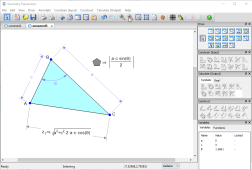# Geometry Expressions

## Explore

See how you can use Geometry Expressions for mathematical modeling, app generation and code generation.

First take a look at how constraint based geometry works, and how it enables you to generate mathematical models.### Getting Started

Videos of Geometry Expressions in action.### Constraints

We explore how constraints work in Geometry Expressions.### Mathematical Modeling

Geometry Expressions is a great mathematical modeling tool because it lets you express problems geometrically and extract algebra from the model.

You can then solve the algebraic model and explore the implications of your solution visually.

Alternatively, you can copy and paste the algebra into a CAS for solution, and paste the result back into Geometry Expressions.

We explore these different approaches in the models below.### App Generation

Geometry Expressions enables you to create rich Math Apps, without any programming. You can create JavaScript apps for embedding in a web page, in fact the tool creates a simple html page incorporating your app. You go from zero to .html in minutes

You can also create apps as Dashboard Widgets for easy incorporation in eBooks using Apple's iBook Author, or as Lua Apps for the TI nSpire.

Take a look at these examples to see how.### Code Generation

With Geometry Expressions, you can draw a diagram and get code generated directly in any one of a dozen different languages.

Cut and paste the code and avoid transcription errors!